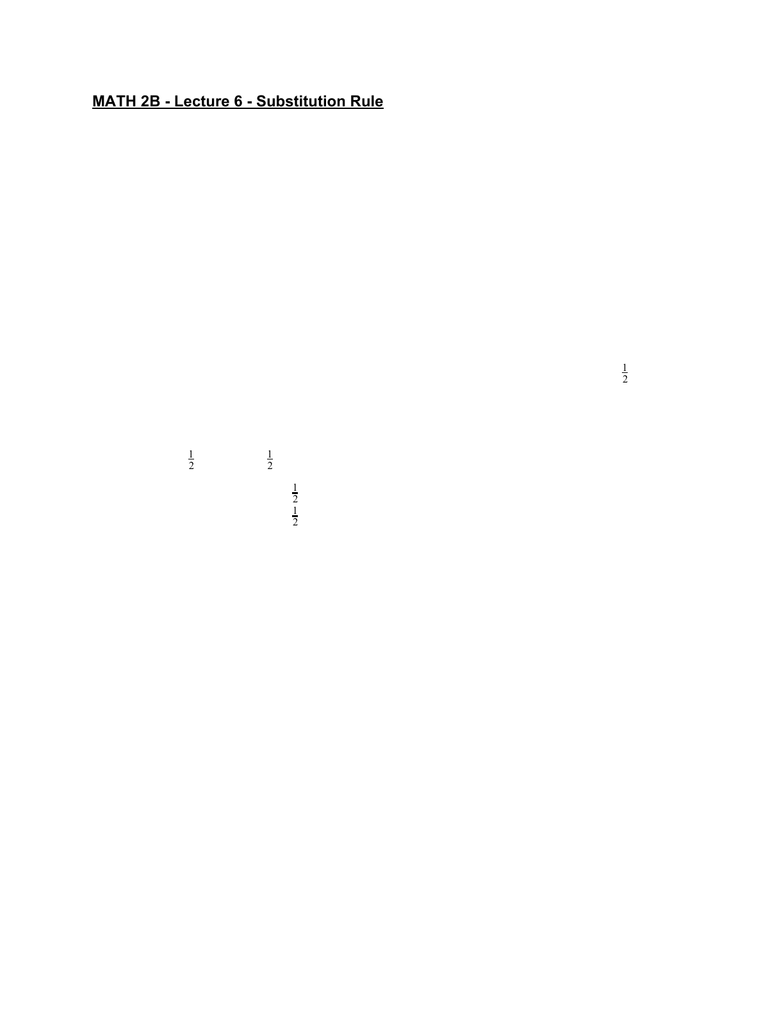Class Notes (1,100,000)
US (460,000)
UC-Irvine (10,000)
MATH (1,000)
MATH 2B (600)
Lecture 6

# MATH 2B Lecture 6: Substitution RulePremium

Department
Mathematics
Course Code
MATH 2B
Professor
ERJAEE, G.
Lecture
6

This preview shows page 1. to view the full 4 pages of the document.MATH 2B - Lecture 6 - Substitution Rule
We begin by considering a single problem.
Ex: in(2x) dx
s
We introduce a new variable u=2x, then the integral becomes:
in(2x) dx
su=2x
in u dx
s
Since dx is not consistent with our new variable u, we then need to translate dx to du.
u=2x du = 2dx dx = ½ du
in(2x) dx
su=2x inu dx
sdx=½ du inu du
s2
1
After introduction the new variable u, and some translations, we end with the integral
inu du (− osu)
2
1
s=2
1c+c
cosu= 2
1+c
cos(2x)= 2
1+cu=2x
This example illustrates the basic idea of u-substitution.
U-Substitution
If u=g(x) is a differentiable function whose range is an interval I and f is
continuous on I, then:
(g(x))g(x)dx
fu=g(x)
(u) du
f
u = g(x) → du = g’(x)dx
That means, if we recognize there is a composite function (“f(g(x))”), it is possible to use
the u-sub to simplify the function, such that we can solve the integral much easier.
To use u-sub, the first step is to recognize the composite function “f(g(x))”
Example:
os(x)2x dx
c2+ 5